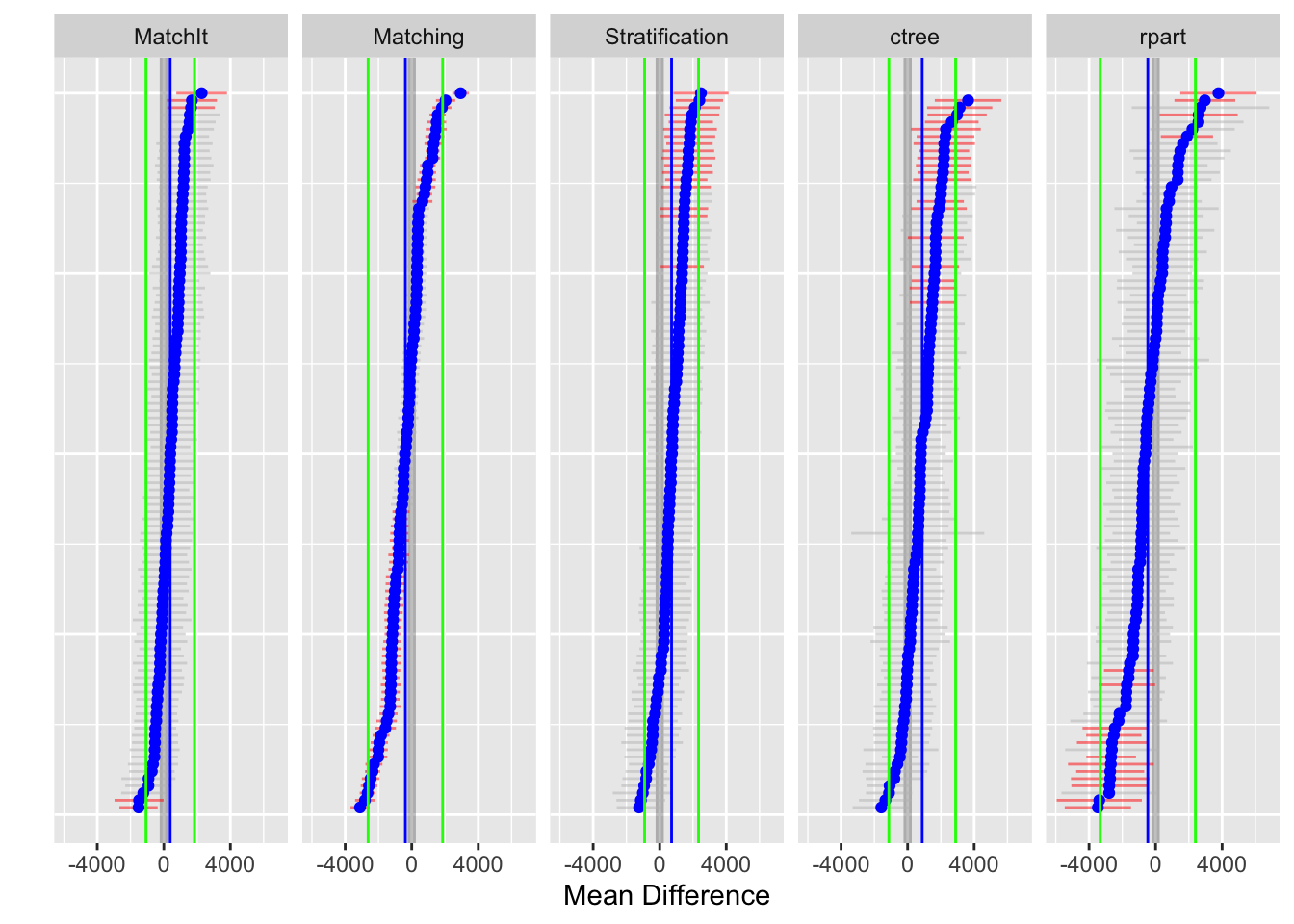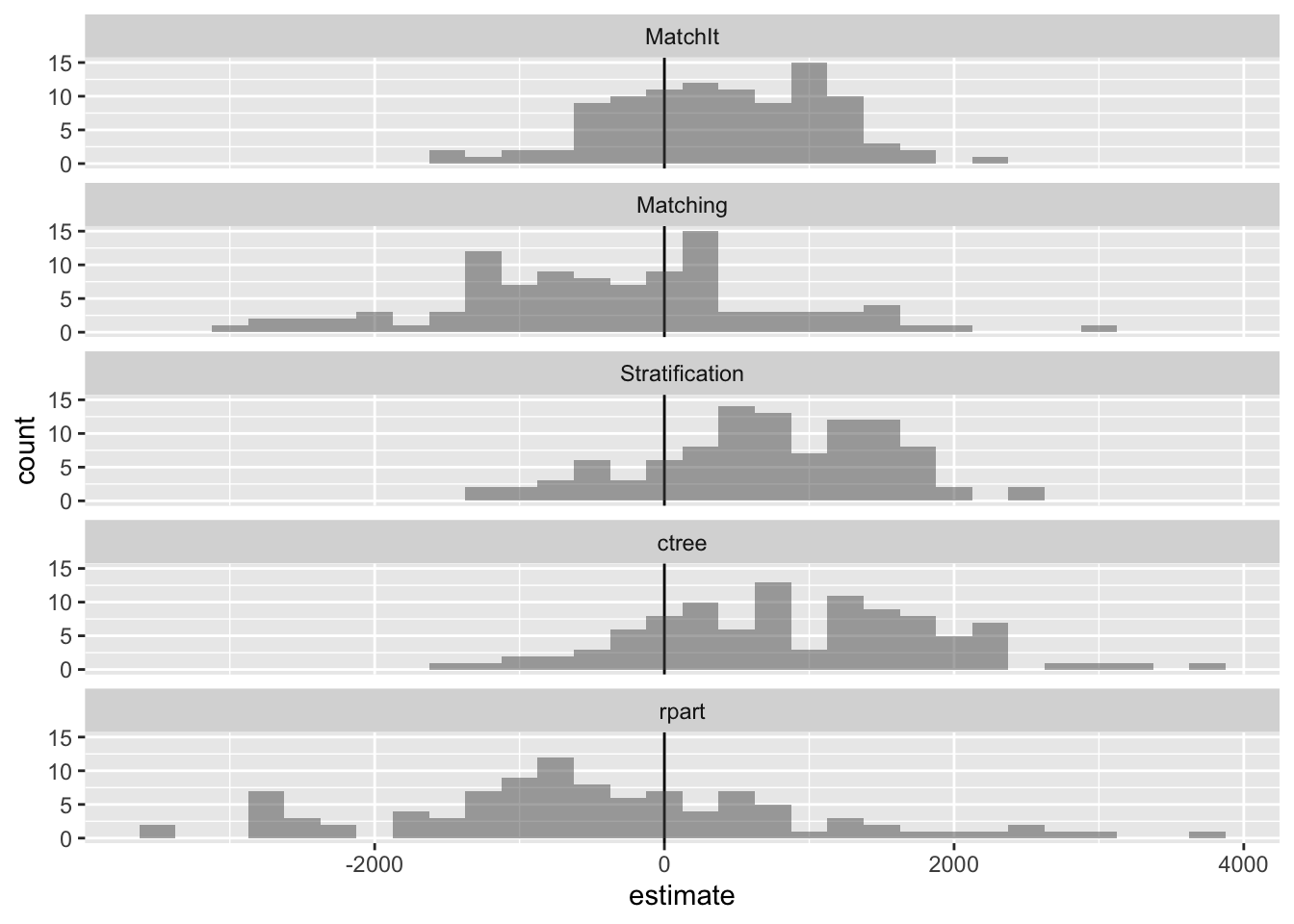Set the number of bootstrap samples. This should be set to at least 100 but kept small to reduce the execution time for CRAN submissions.

``boot.M <- 10``

NOTE: This vignette uses 10 bootstrap samples. It is generally recommended to use at least 100 bootstrap samples or more for final publication.

# Introduction

The latest version of the `PSAboot` package can be downloaded from Github using the `devtools` package.

``devtools::install_github('jbryer/PSAboot')``

The `PSAboot` function will perform the actual bootstrapping. It has a number of parameters for to specify how the bootstrap samples are drawn.

• `Tr` - a numeric (0 for control and 1 for treatment) or logical vector of treatment indicators.
• `Y` - a numeric vector with the outcome of interest.
• `X` - a data frame of covaraites.
• `M` (default is 100) - the number of bootstrap samples to draw.
• `formu` - the formula for estimating the propensity scores in phase I. Note that the dependent variable does not need to be specified as it will be replaced when combining the `Tr` vector and `X` data frame.
• `control.ratio` (default is 5) - This specifies the sample size of control units as a ratio of treatment units. For example, with a default value of 5 and 100 treatment units, this methods will sample 500 control units for each sample, or the number of control units if smaller than 500. When the ratio of treatment-to-control units increases, the range of propensity scores (using logistic regression) shrinks. Randomly selecting a subset of control units often results in wider and better overlapping distribution of propensity scores. See the the `PSranges` function in the `multilevelPSA` package for more information.
• `control.sample.size` (default is 5 times the number of treatment units) - The number of control units to sample for each bootstrap sample. If specified, this overrides the `control.ratio` parameter.
• `control.replace` (default is `TRUE`) - Specify whether random sampling of control units does so with replacement.
• `treated.sample.size` (default is the number of treatment units) - The number of treatment units to sample for each bootstrap sample.
• `treated.replace` (default is `TRUE`) - Specify whether random sampling of treatment units does so with replacement.
• `methods` - A list of functions to perform a propensity score analysis for each bootstrap sample. See the methods section below.
• `parallel` (default is `TRUE`) - Whether the bootstrapping procedure should be run in parallel.
• `seed` - Seed used for the random number generator. If specified, the random seed will be set to `seed + i` where `i` is the current bootstrap sample in (1, M).

Other parameters can be passed to `methods` using the `...` parameter.

## Methods

The `methods` parameter on the `PSAboot` function specifies the different propensity score methods that will be used. Specifically, for each bootstrap sample drawn, each function will be called. This allows for a comparison of methods across all bootstrap samples. Five methods are included, they are:

• `boot.strata` - This method estimates propensity scores using logistic regression and stratifies using quintiles on the propensity scores. Effects within each strata are estimated and aggregated.
• `boot.ctree` - This method creates strata using conditional inference trees vis-a-vis the `ctree` function in the `party` package. Effects within each strata (i.e. leaf node) are estimated and aggregated.
• `boot.rpart` - This method creates strata using classification trees vis-a-vis the `rpart` function. Effects within each strata (i.e. leaf node) are estimated and aggregated.
• `boot.matching` - This method finds matched pairs using the `Match` function in the `Matching` package. A paired dependent sample t-test is used to estimate effect sizes.
• `boot.matchit` - This method finds match pairs using the `matchit` function in the `MatchIt` package. A paired dependent sample t-ttest is used to estimate effect sizes.

### Defining Custom Methods

It is possible to define a custom method. Each method is a function with, at minimum, the following six parameters:

• `Tr` - A logical or integer (0 and 1) vector with treatment indicators.
• `Y` - A numeric vector representing the outcome.
• `X` - A data frame with the covariates.
• `X.trans` - A data frame with factor levels dummy coded.
• `formu` - A formula for estimating propensity scores in phase one.
• `...` - Other parameters passed through from the `PSAboot` function.

Each method must return a `list` with three elements:

• `summary` - This must be a named numeric vector with at minimum `estimate`, `ci.min`, and `ci.max`, however other values allowed.
• `balance` - This must be a named numeric vector with one element per covariate listed in `X.trans` representing a balance statistic. It is recommended, and the implementation for the built-in methods, to use an absolute standardized effect size. As will be shown below, the summary and plotting functions will include an adjusted balance statistic (i.e. effect size) before adjustment for comparison.
• `details` - This can be an arbitrary object, typically the result of the underlying method used.

For example, the `boot.matching.1to3` function below wraps the built-in `boot.matching` method but sets the `M` parameter to 3, thereby performing 1-to-3 matching instead of the default 1-to-1 matching. This framework simplifies the process of using, and comparing, slight variations of different propensity score methods.

``````boot.matching.1to3 <- function(Tr, Y, X, X.trans, formu, ...) {
return(boot.matching(Tr=Tr, Y=Y, X=X, X.trans=X.trans, formu=formu, M=3, ...))
}``````

The `PSAboot` function returns an object of class `PSAboot`. The following S3 methods are implemented: `print`, `summary`, `plot`, `boxplot`, and `matrixplot`.

# Example One: National Work Demonstration and PSID

The `lalonde` (Lalonde, 1986) has become the de defacto teaching dataset in PSA since Dehejia and Wahba’s (1999) re-examination of the National Supported Work Demonstration (NSW) and the Current Population Survey (CPS).

The `lalonde` data set is included in the `MatchIt` package. The crosstab shows that there are 429 control units and 185 treatment units.

``````data(lalonde, package='MatchIt')
table(lalonde\$treat)``````
``````
0   1
429 185 ``````
``````lalonde.formu <- treat ~ age + I(age^2) + educ + I(educ^2) + race +
married + nodegree + re74  + I(re74^2) + re75 + I(re75^2)
boot.lalonde <- PSAboot(Tr = lalonde\$treat,
Y = lalonde\$re78,
X = lalonde,
formu = lalonde.formu,
M = 100,
seed = 2112)``````
``````100 bootstrap samples using 5 methods.
Bootstrap sample sizes:
Treated=185 (100%) with replacement.
Control=429 (100%) with replacement.``````

The `summary` function provides numeric results for each method including the overall estimate and confidence interval using the complete sample as well as the pooled estimates and confidence intervals with percentages of the number of confidence intervals that do not span zero.

``summary(boot.lalonde)``
``````Stratification Results:
Complete estimate = 550
Complete CI = [-1168, 2269]
Bootstrap pooled estimate = 714
Bootstrap weighted pooled estimate = 706
Bootstrap pooled CI = [-907, 2335]
17% of bootstrap samples have confidence intervals that do not span zero.
17% positive.
0% negative.
ctree Results:
Complete estimate = 169
Complete CI = [-1552, 1891]
Bootstrap pooled estimate = 877
Bootstrap weighted pooled estimate = 1091
Bootstrap pooled CI = [-1130, 2883]
19.2% of bootstrap samples have confidence intervals that do not span zero.
19.2% positive.
0% negative.
rpart Results:
Complete estimate = -875
Complete CI = [-2567, 816]
Bootstrap pooled estimate = -470
Bootstrap weighted pooled estimate = 9.9
Bootstrap pooled CI = [-3331, 2392]
16% of bootstrap samples have confidence intervals that do not span zero.
4% positive.
12% negative.
Matching Results:
Complete estimate = -606
Complete CI = [-1296, 83.2]
Bootstrap pooled estimate = -383
Bootstrap weighted pooled estimate = -428
Bootstrap pooled CI = [-2624, 1858]
58% of bootstrap samples have confidence intervals that do not span zero.
16% positive.
42% negative.
MatchIt Results:
Complete estimate = 617
Complete CI = [-781, 2014]
Bootstrap pooled estimate = 384
Bootstrap weighted pooled estimate = 397
Bootstrap pooled CI = [-1072, 1841]
5% of bootstrap samples have confidence intervals that do not span zero.
3% positive.
2% negative.``````

The `plot` function plots the estimate (mean difference) for each bootstrap sample. The default is to sort from largest to smallest estimate for each method separately. That is, rows do not correspond across methods. The `sort` parameter can be set to `none` for no sorting or the name of any `method` to sort only based upon the results of that method. In these cases the rows then correspond to matching bootstrap samples. The blue points correspond to the the estimate for each bootstrap sample and the horizontal line to the confidence interval. Confidence intervals that do not span zero are colored red. The vertical blue line and green lines correspond to the overall pooled estimate and confidence for each method, respectively.

``plot(boot.lalonde)``
``Warning: Removed 1 rows containing missing values (`geom_errorbarh()`).``
``Warning: Removed 1 rows containing missing values (`geom_point()`).``The `hist` function plots a histogram of the estimates across all bootstrap samples for each method.

``hist(boot.lalonde)``The `boxplot` function depicts the distribution of estimates for each method along with confidence intervals in green. Additionally, the overall pooled estimate and confidence interval across all bootstrap samples and methods are represented by the vertical blue and green lines, respectively.

``boxplot(boot.lalonde)``
``Warning: Removed 1 rows containing non-finite values (`stat_boxplot()`).``